﻿ Delta-Sigma A/D Converters in Metrological versus Acoustic ApplicationsElectrical and Electronic Engineering

p-ISSN: 2162-9455    e-ISSN: 2162-8459

2018;  8(1): 24-28

doi:10.5923/j.eee.20180801.03### Delta-Sigma A/D Converters in Metrological versus Acoustic Applications

University of Occupational Safety Management in Katowice, Katowice, Poland

Correspondence to: Tadeusz Sidor, University of Occupational Safety Management in Katowice, Katowice, Poland.
 Email: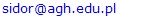Abstract

Delta-Sigma A/D converters are nowadays widely used in digital acoustic systems to record sounds and music. Such converters can also be used in measurement systems to convert analogue voltage signals to digital form. However quality requirements of converters used in measurement systems (Accuracy) and acoustic systems (S/N ratio) are different. The paper attempts to point out the differences in requirements for both kinds of applications.

Keywords: A/D converters, D-S modulators, Oversampling, Quantizing errors, S/N ratio

Cite this paper: Tadeusz Sidor, Delta-Sigma A/D Converters in Metrological versus Acoustic Applications, Electrical and Electronic Engineering, Vol. 8 No. 1, 2018, pp. 24-28. doi: 10.5923/j.eee.20180801.03.

### 1. Introduction

Delta-Sigma Analogue to Digital Converters are nowadays extremely popular in various technical applications, mainly to convert analogue signals of music to digital samples for recording on CD disc, pen-drives or for digital radio signals. Such converters can also be used for various metrological applications. However the metrological applications require different and less rigorous properties of the converter than the one used in acoustic systems.
The Delta-Sigma converters show certain unique feature which make them widely used in various applications. The Delta-Sigma modulators always produce much more samples that it is required to fulfill Shannon theorem i.e. working in oversampling state.
Oversampling by itself spreads quantizing noise along frequency axis and significant part of it is cutoff by digital low pass filter. The one or more integrators within the modulator structure shape the remaining quantizng noise what increases S/N Signal to Noise ratio. Finally last but not least because sampling frequency is much higher than Shannon frequency band the design of analogue antyaliasing filter at the converter input is very simple.
The basic structure of Delta -Sigma converter is presented in figure 1. The input signal is a voltage produced either by measuring transducer or acoustic amplifier. The modulator converts the input analogue signal of voltage to train of one bit (either 0 or 1) pulses, generated by clock of frequency fc = fS, which is much higher than minimum Shannon frequency 2fB required to convert analogue signal, of fB frequency band, into correct digital form. The ratio of fS to 2fB is called the oversampling ratio K (1).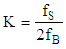(1)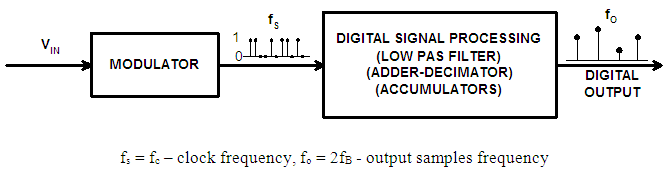Figure 1. Basic structure of Delta-Sigma converter
The output pulses of modulator, which are not uniformly distributed in time, are further digitally processed to convert the low bit samples into multibit samples of required resolution, what is achieved by summing them and simultaneously low pass filtering to reduce quantizing noise.

### 2. Fundamental Structures of Delta-Sigma Converters

In the simplest structure of Delta-Sigma converter the Digital Signal Processing unit can be in fact simple series input, parallel output digital counter. It can be shown that such a counter can serve as both digital low pass filter and decimator .
The origin of Delta-Sigma A/D converter can be traced to synchronous voltage to frequency converter [2, 7] in its classical shape presented in figure 2.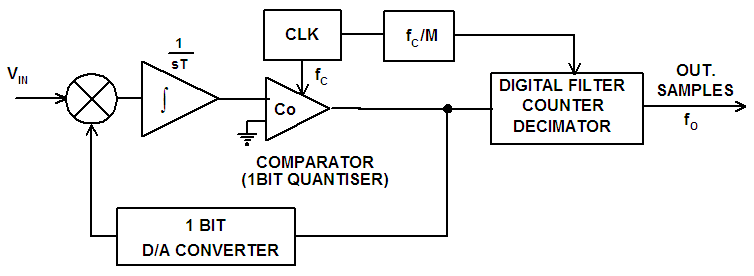Figure 2. Block diagram of A/D converter based on synchronous voltage to frequency converter
Such a structure as presented in fig.2 can also be called Delta-Sigma A/D converter of first order. The converter has two important frequencies fC – clock frequency and simultaneously fS - sampling frequency, fC = fS of the one bit modulator output samples, and fO – converter output multibit samples.
The density of one bit output samples of modulator is proportional to the magnitude of converter input voltage VIN, so if the samples are counted during the fixed time interval determined by M pulses of clock frequency the sum of them, produced at the converter output as N multibit output samples represents the value of the input voltage. The frequency fO of the output samples equals the clock frequency divided by M (2).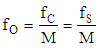(2)
The value of M determines the number N of bits in the output samples as the relation (3) must be fulfilled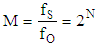(3)
According to Shannon theorem the frequency of converter output samples fO has to be at least twice higher than the highest frequency component of converter input voltage. If the value of fO is than fixed to 2fB the N number of bits in the converter output samples cannot be higher than it is described by relation (3). The M equals than K, the oversampling ratio.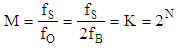(4)
To achieve better resolution N of converter output samples it is necessary either to increase clock frequency or lower the sampling rate. However the clock frequency cannot be increased above technologically determined limits and sampling rate cannot be lower than required for a specific input signal.
It can be than stated that simple Delta-Sigma converters offer a choice between output sampling frequency and resolution of the samples. The number of bits in the output samples can be increased at the cost of lowering sampling frequency.
Another way to achieve higher resolution of converter output samples is to use modulator with multibit comparator at the output. As in the diagram of converter presented in figure 2 the comparator is a one bit device i.e. it produces single bit samples at its output, some more elaborate comparators can be used which can produce multibit samples at the output. The solution requires using also multibit Digital to Analogue converter in the modulator feed-back path. It creates significant problems in practical implementation, as precision of such converter should be better than LSB of the Delta-Sigma converter output samples. Therefore seldom comparators of more than four bit resolution are used. Some manufacturers employ randomization units, which made precision of Digital to Analogue converter less rigorous.
If the achievable resolution of output samples is still not sufficient there are some additional means to increase it. The converter chip manufacturers are very reluctant to reveal how it can be achieved, but some indications point out that parallel decimation might be employed [3, 14, 15].
The parallel decimation arrangement can be illustrated in figure 3.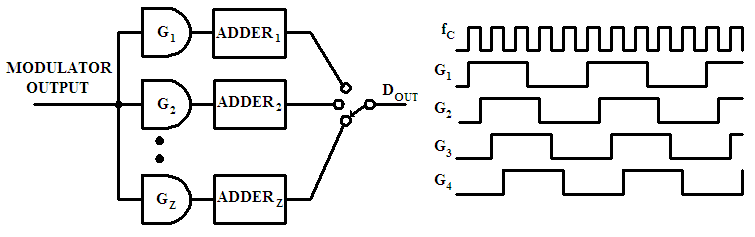Figure 3. The basic arrangement of parallel decimation system
The one or multibit output samples of modulator are simultaneously summed in many adders. Samples are connected to adders through gates G, which are opened to modulator samples in periods shifted by certain number of pulses of modulator clock. The gates are open for the periods sufficient to sum the output samples of modulator to the required final resolution of converter output samples [4, 8].
The resultant resolution of converter output samples i.e. number of bits N of the samples can be achieved through high sampling frequency fS, sufficient oversampling and parallel decimation can be quite high.

### 3. Metrological versus Acoustic Converters Properties

Let us consider example data taken from AD 1871 chip specification. Sampling frequency fS = 13.288 MHz, 256 oversampling rate and 3 bit modulator comparator. It is sufficient to create 11 bit converter output samples with Shannon sampling frequency of 48 kHz. Using just two parallel adders the number of bits can be increased to 12.
It might be sufficient for normal metrological application, but can not be taken for granted for converters used in acoustic systems.
Let us to analyze and compare requirements of converter to be used in measurement systems and acoustic applications.
In metrology transducers and converters accuracy is usually described by accuracy class index i.e. figure which describes in percents transducer limiting error related to its range. When input signal decreases the limiting error proportionally rises. It is common knowledge and when employing such device in measuring circuit care must be taken to set the magnitude of measuring signal as close to the converter range as possible. It usually satisfies the accuracy requirements as typical measuring signals have limited dynamic of 40 dB e.g. i.e.
1: 100. The 12 bit converter distinguish 4096 signal levels, so the quantizing limiting error of ½ LSB is equivalent to 0.012%. Even if the magnitude of signal decreases to 10% of assumed maximum value, the relative errors rises only to 0.12%.
It should be also mentioned that Shannon sampling frequency of 48 kHz is not often needed in normal industrial measurements. If the basic sampling frequency can be lover than the resolution of output samples can be increased.
Completely different situation takes place when converter is used to proceed acoustic signal of music. Typical signal of that kind has dynamic of up to 120 dB i.e. 1: 1000000. This reflects the sensitivity of human ears which can receive sounds from quiet whisper to sounds of heavy metal bands. The errors of converter, in the shape of noise, should not be heard even when the signal is very low. Therefore the accuracy of converters used for digital acoustic systems is usually not expressed as limiting errors, but as signal to noise ratio S/N expressed in dB.
To satisfy proper reception of acoustic signal of music converters used to convert analogue music signal to digital form, the converter should have the signal to noise ratio of similar order as dynamic range of music signal i.e. around 120 dB.
It is not an easy task to achieve such a level, and various methods are used to make signal to noise ratio of A/D converters used for acoustic applications close to the required level.
Fortunately the Delta-Sigma A/D converters have by themselves properties which increase signal to noise ratio: the oversampling, which is always present in Delta-Sigma converters and phenomenon of so called “noise shaping” thanks to the integrator(s) present in the converter feed-back loop (fig. 2).
The basic formulae giving figures of S/N ratio for A/D converter of any kind are given in many papers e.g. [5, 6] and finally are presented in the form (5)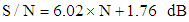(5)
where N – number of bits in the comparator output samples
The Delta-Sigma converters further reduce the noise level because the oversampling causes the quantizing noise to be spread along frequency axis up to fS and further all the frequencies above 2fB are eliminated by the digital low pass filter.
The power of quantizing noise is than decreased by the factor of oversampling K = fs/2fB and finally S/N ratio will rise by the value expressed by (6)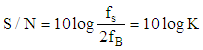(6)
It can be proved  that the integrator in Delta-Sigma converter feed-back loop acts as high pass filter for quantizing noise, removing it from signal frequency band fB and pushing significant part of it into frequency band which, similarly as for oversampling, is eliminated by the digital low pass filter.
Calculating the noise shaping function it can be evaluated that the increase of S/N value can be expressed by (7)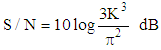(7)
The effects of oversampling for any kind of A/D converter on noise level and signal to noise ratio S/N is illustrated in figure 4 B, and compared with noise level of converter without oversampling in figure 4 A.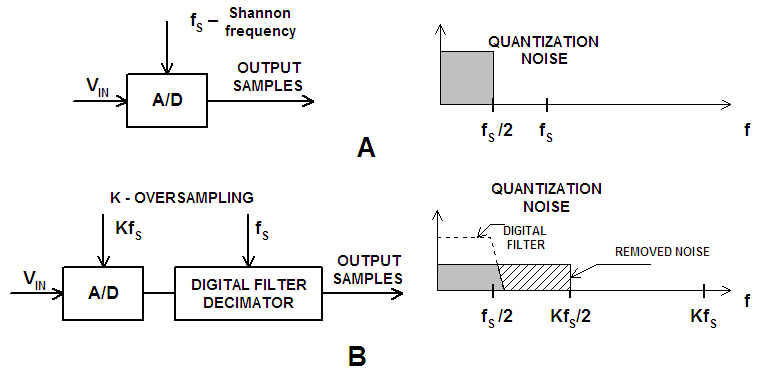Figure 4. The quantizing noise of any kind of A/D converter A, and effect of oversampling on decreasing the noise level B 
In figure 5 the cumulative effects of oversampling and noise shaping on noise level in case of converter with Delta-Sigma modulator is presented.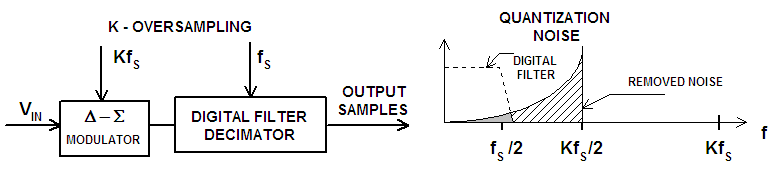Figure 5. The cumulative effect of oversampling and noise shaping for A/D converter with Delta-Sigma modulator on quantizing noise level 
Using formulae (5), (6) and (7) to evaluate Delta-Sigma converter S/N ratio the resultant value are usually greatly overestimated. Among others reasons is one very important. The quantizing noise is basically not uniformely spread along frequency axis. There are some frequencies which are dominant, because Delta-Sigma modulators of first order shows, for certain value of input signals, significant periodical components in the quantizing noise spectrum. The periodical components can be heard in acoustic signals of low magnitude as additional idle tones.
Theoretically the periodical components can be eliminated by dithering of the input signal, but in practical solutions Delta- Sigma modulators of higher order are employed.
The basic circuit diagram of Delta-Sigma modulator of second order is presented in figure 6. The modulator of higher order causes the quantizing noise periodical components to decrease, and simultaneously increases S/N signal to noise ratio.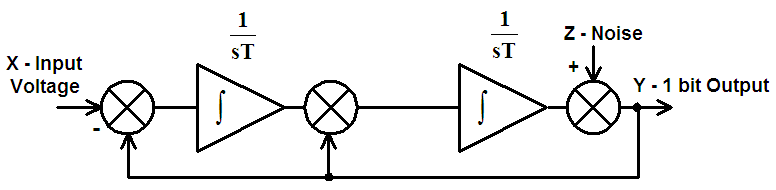Figure 6. Basic circuit diagram of Delta-Sigma modulator of second order
In practical devices Delta-Sigma modulators of up to fifth order are used, although such circuit structure causes its structural instability and integrators have to be replaced by suitable low pass filters.

### 4. Conclusions

In several references [9-12] it is shown that noise shaping technique, implementation of comb-type decimalization output filters and other means which can, by using sigma – delta modulators of higher order, significantly improve signal to noise ratio, resulting in digital acoustic systems of better quality.
There is no doubt that converters with first order delta modulators exhibit a periodical pattern of quantizing error in the output digital words which causes the appearance of idle tones when the converter is used in audio systems, and that the higher order modulators eliminate the problem by providing a kind of input signal dithering.
However if the A/D converters based on sigma –delta modulators are used separately e.g. in measurement systems, it is not the level of noise, but rather the level of the limiting error which is of prime importance.
All the published analysis does not consider the crux of the matter which is the uncertainty of converter digital output due to the quantizing error which is essential in metrological converter applications, but concentrate on pointing out that modulators of higher order are much better as they provide a higher signal to noise ratio. This may lead to the conclusion that higher order delta modulators used in analogue to digital converters somehow reduce the quantizing error of the conversion results.
In order to determine the limiting errors of A/D converters that are used in measurement systems, time domain analysis can be used. This analysis, apart from being a natural tool for such an investigation, also gives physical insight into the complex processes taking place in sigma-delta modulators, particularly those of higher orders.
Somehow the followers of Delta modulation use exclusively frequency analysis and time domain analysis is very seldom used although for metrological purposes it is essential.
As it was mentioned previously A/D converters based on Delta Sigma modulators are today widely employed in acoustic digital systems to convert analogue sound signals into digital form. The digital samples are then recorded e.g. on CD, or transmitted through some kind of network. As digital signals, received or read from a CD, are converted back into an analogue voltage to drive amplifiers and speakers or headphones analysis of such system very often takes into account jointly A/D and D/A conversions which could lead to misunderstandings if this is not clearly specified.

### References

  T. Sidor T, Time domain analysis used to investigate metrological properties of delta-sigma type A/D converters, International Journal of Electronics Letters, Volume 2, Issue 3, July 2014.  U. Tietze, Ch. Schenk, Electronic Circuits – Handbook for Design and Applications, Springer-Verlag 2008.  AN-283 Application Note. Sigma-Delta ADCs and DACs. Analogue Devices, One Technology Way. P.O. Box 9106, Norwood, Massachusets 02062-9106, 617/329- 4700.  T. Sidor T, Elimination of analog impairments problems in parallel interleaving sigma delta converters, Electrical and Electronic Engineering 6(2) 2016.  W. Kester, Taking the Mystery out of the Infamous Formula, "SNR = 6.02N + 1.76dB," and Why You Should Care. Analog Devices Tutorial MT- 001.  Uwe Beis. An Introduction to Delta Sigma Converters: www.beis.de.  Walt Kester and James Bryant, Voltage –to-Frequency Converters, Analog Devices. Tutorial MT – 028.  G. Manganaro, D. Robertson, Interleaving ADCs: Unraveling the Mysteries, Analog Dialogue 49-07, July 2015.  Sangil Park: Principles of Sigma-Delta Modulation for Analog-to-Digital Converters. Motorola APR8/D, Rev.1, http://www.numerix-dsp.com/appsnotes/APR8-sigma-delta.pdf.  Maxim Application note No 1870: Demystifying Delta–Sigma ADCs., https://www.maximintegrated.com/en/app-notes/index.mvp/id/1870.  M. Pervez, Aziz, Henrik V. Sorensen and Jan Van Der Spiegel: An Overview of Sigma Delta Converters. IEEE Signal Processing Magazine Vol. 13, Jan/1996.  W. Kester, ADC Architectures IV: Sigma-Delta ADC Advanced Concepts and Applications, Analog Devices Tutorial MT-023.  T. Sidor, Metrological properties of A/D converters utilizing higher order sigma-delta modulators compared with A/D converters with modulators of first order, Metrology and Measurement Systems, Vol 21, NR 1, 2014.  A. Eshraghi, T. S. Fiez, A Comparative Analysis of Parallel Delta–Sigma ADC Architectures, IEEE Transactions on circuits and systems, Vol. 51, no. 3, march 2004.  B. Razawi, Design Considerations for Interleaved ADCs, IEEE Journal of Solid-state Circuits, Vol. 48, no. 8, August 2013.